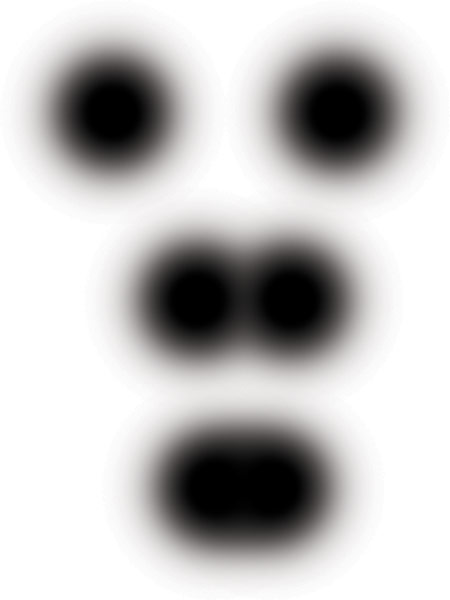# The Airy Disc and Resolution

back

The distance between two Airy discs where they are still considered to be resolved separately is the radius of the disc - also called the Raleigh criterion. A smaller Airy disc means a smaller disc radius and a higher resolution. This distance is somewhat arbitrary as there is still a small amount of contrast remaining in the space between the discs at the Raleigh criterion. If the discs are moved any closer, the remaining contrast between the two objects will completely disappear. The point where all contrast is lost between the adjacent discs is called the Sparrow criterion and is the absolute limit of resolution. The Raleigh criterion is the most commonly used measure of resolution:

Airy disc radius = 1.22 * N * light wavelength ( N = aperture size, light wavelength is commonly 546 or 550 nm or 0.550 um, a wavelength of green)

The Airy disc diameter is just double the radius. The Sparrow criterion is expressed as:

D = 0.947 * N * light wavelength (about 80% of the Raleigh criterion)

The resolution limit of a lens is really just a measure of the diffraction in the system. We will assume that the lens doesn't have significant aberrations limiting things further. To lessen the diffraction, you can do one of two things: 1) enlarge the aperture (lower f/number), 2) lower the wavelength of the light (blue should produce more resolution than red will). The Airy disc as described in the previous entry is the smallest point of light that can be focused by a lens at a particular aperture and represents the center of a diffraction pattern. The size of the disc is a direct determinant of image resolution. The resolution of a lens is defined as the closest that two objects can be to each other while still being separately resolved by the lens. The two objects in question are two Airy discs. As the two discs get closer and closer together they will start to merge and at a certain point the separation between them will be lost.